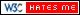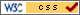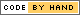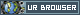# Digit Recognition

## Introduction

In this project, we implement a self-learning digit recognition algorithm to determine the input given by a user using the piTFT touch interface. This project also explores the various techniques available to speed up the execution of the program.

## Objective

This project explores two major aspects of computer programing - machine learning and parallel processing. The first objective of this project is to implement a self learning digit recognition algorithm which takes input from the user, compares it against an existing data-set and returns a digit the input resembles. Since the comparison has to be done across dataset from 10 numbers (0-9), the task is distributed accross the processors to ensure minimum execution time.

## Design

The project was divided into three phases:

### Phase 2: Implementation of K-NN Algorithm

The KNN algorithm: k – nearest neighbor is a classifying algorithm that is used in handwriting recognition. It is a machine learning algorithm. In this algorithm, the input data is classified into classes based on its distance from a set of data that has already been classified. Since the array being operated upon is a one-dimensional array, the distance between the testing (input) data and training data was calculated using Manhattan distance.

### Phase 3: Parallel Processing

The KNN algorithm has high of parallelism which can be exploited using Parallel processing. Both C and python code was optimized to reduce the overall runtime and improve the system performance.

## Results

The Python program developed for digit recognition was tested repeatedly. The input digits were varied and drawn on different parts of the screen. The accuracy of the program increased steadily as the learning data-set of each digit kept growing. In addition, the multi-processing optimization ensured that the total execution time of compute intensive part of the program remained well below one second.

One key difference between C and Python implementation was the size of the database. Python used a database of around 100 training sets for each digit whereas C program used 1800. Hence, the total execution time of Python implementation and C++ implementation couldnot be compared directly.

Following are the results for the C implementation: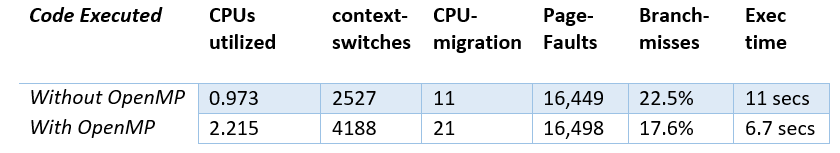C++ Results

The above table summarizes the performance measurement using C++. It is observed that the execution time decreased for optimized version since more than 1 core is being utilized. But however context-switches and CPU-migrations are higher as the program is continuously switched back and forth between multiple threads. The page fault is almost the same since the entire training data set is accommodated in the main memory. Also since the code uses multiple backward edges due to for loops and it has high level of parallelism, the total number of branch misses can be reduced by exploiting thread level parallelism (TLP).

## Conclusion & FutureScope

The self-learning digit recognition was successfully implemented using Python and a performance improvement of 3x was obtained using multi-processing. The C++ implementation was also optimized using OpenMP and performance improvement of 2x was observed.

The scope of digit-recognition program can be further extended to character and other symbol recognition. Similar self-learning KNN algorithm can be utilized to accurately predict the input given using piTFT screen. In addition, this program can also be utilized in developing mobile applications for gesture recognition.

Future directions for this project would be to develop a C/ python interfacing to leverage the pygame module for pi-TFT user input as well as achieve an overall better runtime in C compared to python implementation. Possible extensions for the current project would be to implement the Python calling C function successfully.

## Code Appendix

### Self learning digit recognition program

```# Developed by Mayank Sen Sharma (ms3273) and Siddharth Mody (sbm99)
# for course Project of ECE5990
# Note: This program implements self learning digit-recognition algorithm
#       This program required 10 learning files in its execution directory

import pygame
import operator
from multiprocessing import Process, Queue
import multiprocessing
import os
import time

os.putenv('SDL_FBDEV', '/dev/fb1')
os.putenv('SDL_MOUSEDRV', 'TSLIB')
os.putenv('SDL_MOUSEDEV', '/dev/input/touchscreen')

def get_points():
pygame.init()
screen = pygame.display.set_mode((320, 240))
clock = pygame.time.Clock()

x = 0
y = 0
points = []

while True:

pressed = pygame.key.get_pressed()

for event in pygame.event.get():

if event.type == pygame.QUIT:
return

if event.type == pygame.MOUSEMOTION:
if event.buttons:
# if mouse moved, add point to list
position = event.pos
points = points + [position]
points = points[-500:]

if event.type == pygame.MOUSEBUTTONUP:
pygame.quit()
return points

screen.fill((0, 0, 0))

# draw all points
i = 0
while i < len(points) - 1:
drawLineBetween(screen, i, points[i], points[i + 1], radius)
i += 1

pygame.display.flip()

clock.tick(24)

# Function to draw the figure on screen
def drawLineBetween(screen, index, start, end, width):
color = (255, 255, 255)

dx = start - end
dy = start - end
iterations = max(abs(dx), abs(dy))

for i in xrange(iterations):
progress = 1.0 * i / iterations
aprogress = 1 - progress
x = aprogress * start + progress * end
y = aprogress * start + progress * end
pygame.draw.circle(screen, color, (int(x), int(y)), width)

# computing K nearest neighbor
def k_nearest_neighbor(start, step, q):

Knn = [[300 for x in range(4)] for x in range(10)]
num = start

for x in range(step):
f = open("data_{}.dat".format(num),"r")

while line:
# code for converting string to list
line = line.strip('[')
line = line.strip(']\n')
line = line.split(",")
data = [int(e) for e in line ]

# Distance between test and training set
dist =  sum(map(operator.xor, Matrix, data))

index = 0
for x in Knn[num]:
if dist < x:
Knn[num][index] = dist
break
index += 1

num += 1
f.close()

q.put(Knn)

# Main part of program

points = get_points()
# screen size 320 x 240
# dividing by a factor of 16
Matrix = [0 for x in range(20*15)]

i = 0
while i < len(points) - 1:
x = int(points[i]/16)
y = int(points[i]/16)
i += 1
Matrix [ x + 20*y ] = 1

# initialise K-nearest neighbor array
Knn = [[300 for x in range(4)] for x in range(10)]

# getting number of CPUs
num_cpus = multiprocessing.cpu_count()

# for distribution of distance calculation
step = 10/num_cpus;

# Initializing queuess for function return
ques = [Queue() for i in range(num_cpus)]

# creating multiple processes
proc = [Process(target = k_nearest_neighbor, args=(i*step, step, ques[i])) for i in range(num_cpus - 1)]

proc.append(Process(target = k_nearest_neighbor, args=((num_cpus - 1) * step, 10 - (num_cpus - 1)* step, ques[num_cpus - 1])))

start_time = time.time()

# Starting processes
for i in range(num_cpus):
proc[i].start()

# storing return values from processes
knn = [0 for x in range(num_cpus)]

for i in range(num_cpus):
knn[i] = ques[i].get()

print("\n--- %s seconds ---\n" % (time.time() - start_time))

for i in range(num_cpus - 1):
Knn[i*step:(i+1)*step] = knn[i][i*step:(i+1)*step]

Knn[(num_cpus - 1) * step : 10 ] = knn[num_cpus - 1] [(num_cpus - 1) * step : 10 ]

# Voting function
num           = 0
predicted_num = 0
minimum       = 1200

while num < 10:

total = sum(Knn[num])
print str(num) + " : " +str(total)
if total < minimum:
minimum       = total
predicted_num = num
num += 1

print "My Prediction: " + str(predicted_num)

a = True

while a:

check = raw_input('Is my prediction correct? (y/n):')

if (check == "y"):
print "YES!"
a = False

elif (check == "n"):
predicted_num = input('Please enter the number: ')
a = False

else:
print "You entered wrong input, please try again..."

if Knn[predicted_num] != 0:
with open("data_{}.dat".format(predicted_num), "a") as myfile:
myfile.write(str(Matrix) + "\n")
```

## Source code

Python and C++ source code available below: